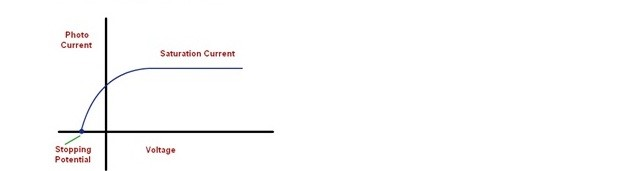Categories

# ENERGY QUANTIZATION (Photoelectric Effect)

INTRODUCTION

In 1887, photoelectric effect was invented by the scientist H. Hertz. When we passing a light into a material, the material should emit an electrons. This effect is called as Photoelectric effect. Some of the rays produced in Photoelectric Effect are x-rays, ultraviolet rays and γ-rays.

#Einstein’s says that every photon or a quantum of light has an energy value hν. Electrons are located inside the surface of the metal. There may be some amount of work W should be required for an electron to come out of the metal. If the transferred energy is high comparing the internal attractions then the electrons gets liberated. In this the one photon can release a one electron. This is nothing but the Photoelectric Effect. Let us study more about the Photoelectric effect in this section.

## Photoelectric effect slides: How Einstein became famous!

https://www.slideshare.net/slideshow/embed_code/key/526acVQzpVvxMOPhotoelectric Effect from Kendriya Vidyalaya Sangathan

# Photoelectric Effect Definition

It is the phenomenon of emission of electrons from the surface of metals when the radiations of suitable frequency and suitable wavelength fall on the surface of the metal.

The following parameters are related to Photoelectric effect:

Photoelectric Current

Stopping Potential

Threshold Energy

Work Function

The emitted electrons are called photo electrons and the current so produced is called as the Photoelectric Current. The intensities of photo electrons vary with material.

The Stopping Potential is the potential difference applied to stop the electrons from being ejected from the surface when the light falls on it.

The Threshold Frequency is defined as the minimum frequency of incident light required for the photo electric emission. It is denoted by ν.

Work Function is the minimum amount of energy necessary for the photo electric emission to start. It is denoted by ϕ0.

Photoelectric Effect Equation

The Einstein equation describes the phenomena of photoelectric effect but the validity of this equation is only up to two regions, one is visible light and second is for ultraviolet light.

The energy of photon is equal to energy required to remove electron + kinetic energy of the emitted electron.

E = W + K.E.

where E = Energy of photon
W = Work function
K.E = Kinetic Energy.

Energy of photon, E = h ν …………………….. (a)

Where h = Planck’s constant

ν = frequency of photon incident light or

So ν = W + K.E …………………………… (b)

The work function W is defined as the minimum energy needed to remove an electron from the surface of a given metal. It is equal to h ν0.
K.E= This is the kinetic energy (maximum) of emitted electrons.

K.E = 1/2 mv2.

Here ν0 is the threshold frequency.

m = rest mass of the emitted electron

v = The speed or velocity of the emitted electron.
Work function or threshold energyϕ ):
The minimum energy of incident photon below which no ejection of photon electron from a metal surface will take place is known as work function of threshold energy for that metal.

ϕ = h ν0 = hC/λ0

Work function is the characteristic of a given metal. If E is the Energy of incident photon then

1. If E < ϕ, no photo electric effect will take place.
2. If E = ϕ, photoelectric effect will take place but kinetic effect of ejected photo electron is zero.
3. If E > ϕ, photo electric effect will take place along with possession of kinetic energy by ejected electron.

Photoelectric Effect Experiment

The Phenomenon was discovered by Hertz and was experimentally proved by Thomson and Millikan. Einstein added his photoelectric equation to support the phenomenon. The phenomenon is basically about light energy (photo) converting into electrical energy.

The Apparatus consists of an evacuated quartz tube having photosensitive plate called emitter A and collector B. Plate A is connected to the negative terminal and plate B is connected to the positive terminal of a battery via rheostatR. The potential difference between the plates AB can be adjusted by changing the value of the rheostat. When light of suitable wavelength is incident on the plate A then electrons are emitted and reaches plate B, thus measurable current flows through the circuit.

Factors on which Photoelectric effect depends :
The amount of current flow (number of electrons) and the kinetic energy of the emitted electrons depend upon :

1. Applied Potential difference between the plates:
For a given photo metal if the frequency and intensity of incident light kept constant and if the potential difference between the plates is increased then photo current also increases until it reaches a maximum (saturated) value. If the terminals are reversed and we increase the potential difference gradually then photo current decreases and at one point it becomes zero. This point where the current is zero is termed as Stopping Potential.2. Intensity of incident radiation:For a given photo metal if the frequency and applied voltage is kept constant with increasing intensity then we observe that photo current increases with increases in intensity with out change in the stopping potential.
3. Material of the Photo metal:
The experiment is repeated for different photo metal and their threshold frequencies are noted. The variation of frequency versus stopping potential is graphed and noticed that threshold frequency varies from metal to metal.